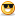menu

# Thread: BDO New World | Server BR | Easy Server | 20+ Online

1. ## BDO New World | Server BR | Easy Server | 20+ Online

Black Desert New World

Rates:
[Version 491]

Monster drop rate: x35
Fishing drop rate: x10
Collection drop rate: x20
Steal drop rate: x10
Tendency Exp rate: x10
Horse Exp rate: x200

Exp rate: x120
Skillexp rate: x200
GuildSkillexp rate: x200
Fitness exp rate: x50
Life exp rate: x10
Explore exp rate: x10

Enchantment Rates:
[Armors:]
= +1 to +8 = 100%
= +8 to +9 = 90%
= +9 to +10 = 80%
= +10 to +11 = 75%
= +11 to +12 = 70%
= +12 to +13 = 69% (Max.FS = 3 = 72%)
= +13 to +14 = 68% (Max.FS = 7 = 75%)
= +14 to +15 = 60% (Max.FS = 12 = 72%)
= +15 to PRI = 58% (Max.FS = 20 = 78%)
= PRI to DUO = 56% (Max.FS = 20 = 76%)
= DUO to TRI = 10% (Max.FS = 25 = 35%)
= TRI to TET = 4% (Max.FS = 25 = 29%)
= TET to PEN = 2% (Max.FS = 25 = 27%)

[ Weapons: ]
= +1 to +8 = 100%
= +8 to +9 = 90%
= +9 to +10 = 80%
= +10 to +11 = 75%
= +11 to +12 = 70%
= +12 to +13 = 69% (Max.FS = 3 = 72%)
= +13 to +14 = 68% (Max.FS = 7 = 75%)
= +14 to +15 = 60% (Max.FS = 12 = 72%)
= +15 to PRI = 50% (Max.FS = 20 = 70%)
= PRI to DUO = 45% (Max.FS = 25 =65%)
= DUO to TRI = 4% (Max.FS = 30 = 34%)
= TRI to TET = 2,5% (Max.FS = 30 = 32,5%)
= TET to PEN = 1% (Max.FS = 30 = 31%)

[Accesory:]
= PRI = 60% (Max.FS = 10 = 70%)
= PRI to DUO = 30% (Max.FS = 10 = 40%)
= DUO to TRI = 10% (Max.FS = 10 = 20%)
= TRI to TET = 10% (Max.FS = 10 = 20%)
= TET to PEN = 7% (Max.FS = 10 = 17%)

= Sucess FS = 1 %

Any doubts, look for me
[Head Admin] DrPsykoNew World :Reply With Quote

2. kkkkkkkkk j? fechou?Reply With Quote

3. kkkkkkkkk j? fechou?
Não, estamos migrando para a 661vReply With Quote

4. update discord linkReply With Quote

5. Update discord link! "Manda o link novo do discord manin esse ai ja elvis aguardando"Reply With Quote

• ### About Us

OwnedCore- World of Warcraft Exploits, Hacks, Bots and Guides
All times are GMT -5. The time now is 08:20 PM. Powered by vBulletin® Version 4.2.3